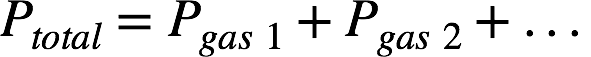# Problem: A mixture consisting of 7.0 g of CO and 10.0 g of SO 2, two atmospheric pollutants, has a pressure of 0.33 atm when placed in a sealed container. What is the partial pressure of CO?

###### FREE Expert Solution

Recall: Dalton’s law states that the total pressure of a mixture of unreacting gases is the sum of the partial pressures of the component gases.The partial pressure of a gas in the mixture is the product of the total pressure of the mixture and the mole fraction of the gas:We're given the mass of CO and SO2 and the total pressure of the mixture. We first need to calculate the mole fraction of CO and then we can calculate the partial pressure of CO.

80% (478 ratings)###### Problem Details

A mixture consisting of 7.0 g of CO and 10.0 g of SO 2, two atmospheric pollutants, has a pressure of 0.33 atm when placed in a sealed container. What is the partial pressure of CO?